Bittensor: A Peer-to-Peer Intelligence Market

YUMA RAO00/ Abstract

As with other commodities, markets could help us efficiently produce machine intelligence. We propose a market where intelligence is priced by other intelligence systems peer-to-peer across the internet. Peers rank each other by training neural networks which learn the value of their neighbors. Scores accumulate on a digital ledger where high ranking peers are monetarily rewarded with additional weight in the network. However, this form of peer-ranking is not resistant to collusion, which could disrupt the accuracy of the mechanism. The solution is an incentive mechanism which maximally rewards honestly selected weights, making the system resistant to collusion of up to 50 percent of the network weight. The result is a collectively run intelligence market which continual produces newly trained models and pays contributors who create information theoretic value.

0.1/ Introduction

The production of machine intelligence has come to rely almost entirely on a system of benchmarking, where machine learning models are trained to perform well on narrowly defined supervised problems. While this system works well for pushing the performance on these specific problems, the mechanism is weak in situations where the introduction of markets would enable it to excel. For example, intelligence is increasingly becoming un-tethered from specific objectives and becoming a commodity which is (1) expensively mined from data (Schwartz et al. ), (2) monetarily valuable (OpenAI ), (3) transferable (Devlin et al. ), and (4) generally useful (Radford et al. ). Measuring its production with supervised objectives does not directly reward the commodity itself and causes the field to converge toward narrow specialists (Chollet ). Moreover, these objectives (often measured in uni-dimensional metrics like accuracy) do not have the resolution to reward niche or legacy systems, thus what is not currently state of the art is lost. Ultimately, the proliferation of diverse intelligence systems is limited by the need to train large monolithic models to succeed in a winner-take-all competition. Standalone engineers cannot directly monetize their work and what results is centralization where a small set of large corporations control access to the best artificial intelligence (OpenAI ).

A new commodity needs a new type of market1 . This paper suggest a framework in which machine intelligence is measured by other intelligence systems. Models are ranked for informational produc- tion regardless of the subjective task or dataset used to train them. By changing the basis against which machine intelligence is measured, (1) the market can reward intelligence which is applicable to a much larger set of objectives, (2) legacy systems can be monetized for their unique value, and (3) smaller diverse systems can find niches within a much higher resolution reward landscape. The solution is a network of computers that share representations with each other in a continuous and asynchronous fashion, peer-to-peer (P2P) across the internet. The constructed market uses a digital ledger to record ranks and to provide incentives to the peers in a decentralized manner. The chain measures trust, making it difficult for peers to attain rewards without providing value to the majority. Researchers can directly monetize machine intelligence work and consumers can directly purchase it.

01 Model

We begin with an abstract definition of intelligence Hinton et al.  in the form of a parameterized function$\ y = f(x) \:$trained over a dataset$\ D = [X, Y] \:$to minimize a loss$\ {\mathcal{L}} = E_{D}[Q(y,f(x))] \:$Our network is composed of n functions$\ F = f_{0}, ..., f_{j}, ...f_{n} \:$’peers’ where each is holding zero or more network weight$\ S = [s_{i}] \:$’stake’ represented on a digital ledger. These functions, together with losses and their proportion of stake, represent a stake-weighted machine learning objective$\sum_{i}^{n} {\mathcal{L}}_{i} * s_{i} \:$Figure 1 / Peer functions with losses$\ {\mathcal{L}}_{i} \:$and unique datasets$\ D_{i} \:$

Our goal is the distribution of stake I, as incentive, to peers who have helped minimize the loss- objective (Figure-1), and importantly, in such a way that, it is difficult for a small proportion of stake to collude as a means to maximize their distribution in the network without minimizing the loss (Figure-3).

## (1)

$\ S_{t+1} = S_{t} + \tau I$

In this paper, we suggest this can be achieved through peer-ranking, where peers use the outputs of others$\ F(x) = [f_{0}(x)...f_{n}(x)] \:$as inputs to themselves$\ f(F(x)) \:$and learn a set of weights$\ W = [w_{i,j}] \:$where peer i is responsible for setting the i th row through transactions on a digital ledger.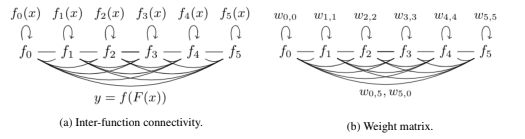Setting weights using an fishers information pruning score LeCun et al. ; Yu et al.  in the ranking calculation,$\ R = W^{T} \cdot S \:$achieves an idealized scoring where each peer’s incentive is equivalent to its pruning score: the cost in entropy towards$\sum_{i}^{n} {\mathcal{L}}_{i} * s_{i} \:$induced by removing it from the network.

## (2)

$\ r_{i} \approx \frac{1}{n} \sum_{j}^{n} \sum_{x \in D_{j}} \Delta F^{T}(x)_{i} \cdot H(Q_{j}(x)) \cdot \Delta F(x)_{i}$

However, this approach is not resistant to collusion, where peers vote for themselves, notably instead of using (2), and set weights to enhance their own inflation at the expense of the network(Figure-3). This attack is trivial since the digital ledger cannot audit the parameters of each model, only the inter-model weights W.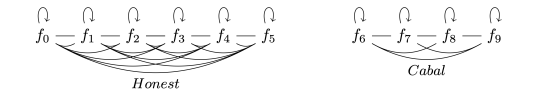Figure 3 / Disjoint cabal: peers in the right sub-network only vote for themselves.

02 Incentive

We extended the naive ranking method to evade collusion with an ’incentive’ function$\ I(W, S) \:$which limits reward to peers which have not reached consensus in the network. Assuming no group of peers holds more than the majority of stake in the system, then peers can only attain inflation by working to attract votes from the majority: a core assumption in many decentralized system like Bitcoin. Reintroducing our terms, our incentive mechanism requires a stake vector S and a set of weights W where rows are inter-peer rankings. We also infer a trust matrix T from the weights, where$\ t_{i,j} = 1 \:$if and only if there is a non-zero edge between peer i and j.

## (3)

$\ W = \begin{bmatrix} w_{0,0} & w_{0,1} & w_{0,2} & w_{0,3} \\ w_{1,0} & w_{1,1} & w_{1,2} & w_{1,3} \\ w_{2,0} & w_{2,1} & w_{2,2} & w_{2,3} \\ w_{3,0} & w_{3,1} & w_{3,2} & w_{3,3} \end{bmatrix} S = \begin{bmatrix} s_{0} \\ s_{1} \\ s_{2} \\ s_{3} \end{bmatrix} T = \begin{bmatrix} t_{0,0} & t_{0,1} & t_{0,2} & t_{0,3} \\ t_{1,0} & t_{1,1} & t_{1,2} & t_{1,3} \\ t_{2,0} & t_{2,1} & t_{2,2} & t_{2,3} \\ t_{3,0} & t_{3,1} & t_{3,2} & t_{3,3} \end{bmatrix}$

We define peers who have reached ’consensus’ as those with non-zero edges from more than 50 percent of stake in the network. (This is simply the normalized values of$\ (T^{T} \cdot S) > 0.5$). To ensure the mechanism differentiable we define this computation using the continuous sigmoid function. The sigmoid produces a threshold-like scaling which rewards connected peers and punishes the non-trusted. The steepness and threshold point can be modulated through a temperature ρ and shift term κ.

## (4)

$\ C = \sigma (\rho (T^{T}S - \kappa))$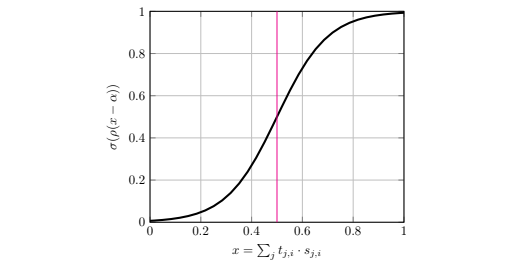Figure 4 / Consensus function$\ c_{i} = \sigma(\rho \sum_{j}^{n} t_{j,i}s_{j} - \kappa) \:$with temperature ρ = 10 and shift κ = 0.5. The activation takes the trust scores and produces an exponential scaling up to our inflection point where a peer is connected to the majority.

We use the consensus term to scale the original rankings. As peers attain more weight in the network they increase their inflation exponentially up to 0.5. In section 10 we show how this ensures that the larger of two competing sub-graphs comes to own an exponentially larger proportion of the network through inflation.

## (5)

$\ I = R \cdot C$

03 Bonds

This consensus described above protects against naive collusion by making it difficult for small groups to achieve inflation. However, it does not provide a incentive for correctly selecting weights. We introduce these incentives by adapting the inflation mechanism with a speculation based reward in the form of ’bonds’ B. Here,$\ b_{i,j} \in B \:$is the proportion of bonds owned by peer i in peer j.

## (6)

$B = \begin{bmatrix} b_{0,0} & b_{0,1} & b_{0,2} & b_{0,3} \\ b_{1,0} & b_{1,1} & b_{1,2} & b_{1,3} \\ b_{2,0} & b_{2,1} & b_{2,2} & b_{2,3} \\ b_{3,0} & b_{3,1} & b_{3,2} & b_{3,3} \end{bmatrix}$

Bonds accumulate at each step similarly to token inflation where$\ \Delta B = W \cdot S \:$In this way, peers accumulate bonds in the peers they rank, thus ’bonding’ themselves to those that they are connected to.

## (7)

$\ B_{t+1} = B_{t} + W \cdot S$

Using the B bond matirx, the chain redistributes the normal incentive scores$\ \Delta S = B^{T} \cdot I \:$Like market based speculation on traditional equities, the peers that have accumulated bonds in peers that others will later value attain increased inflation themselves. Thus it makes sense for peers to accumulate bonds in peers which it expects to do well according to other peers with stake in the system - thus speculating on their future value. Finally, we adapt this mechanism slightly to ensure peers attain a fixed proportion of their personal inflation. For instance, 50 percent,$\ \Delta S = 0.5B^{T}I + 0.5I. \: \Delta S \:$becomes the mechanism step update with determines network incentives across the n peers.

## (8)

$\ S_{t+1} = S_{t} + \tau \Delta S$

04 Reaching Consensus

The incentive function in section 2 rewards highly trusted peers, however, it may not solve the collusion problem if the honest nodes do not reach consensus. Notably loose, unused stake or incorrectly set weights will detract from the inflation proportion of honest peers in comparison to a colluding sub-network. The honest network, although holding more stake, may not gain enough inflation to overshadow its adversary. The dishonest sub-graph need only attain enough inflation to compete with its largest competitor, not to entirely dominate the network.

This attack is possible when the majority of token inflation is being distributed towards peers which are non-majority-trusted in the graph. The chain can measure this through a ’loss term’$\ {\mathcal{L}} = -R \cdot (C - 0.5) \:$(Figure 7). The term is negative iff the majority of inflation is being distributed towards peers with more than 0.5 consensus. The chain uses the loss calculation as a peg. By increasing the number of weights the average miner sets across the network the chain can ensure consensus.Figure 5 / The left network has low consensus$\ {\mathcal{L}} > 0 \:$The system is not resistant to a cabal with less than 50 percent of the stake. The chain increases the number of edges set by peers until$\ {\mathcal{L}} < 0\:$. At this point the majority of inflation flows to peers with majority consensus.

05 Running the Network

The steps to run a peer in the network are:

1. The peer defines its dataset$\ D_{i} \:$
2. At each training iteration, the peer conditionally broadcasts batches of examples from$\ D_{i} \:$to its peers x = [batch_size,sequence_length, input_size].
3. The responses$\ F(x) = [...f_{j}(x)...] \:$– each of common shape$\ f_{j}(x)\:$= [batch_size, sequence_length, output_size] – are joined using the gating function and used as input to the local model$\ f_{i} \:$
4. Comparison against the target labels produces a loss-gradient$\ \frac{\partial \mathcal{L}}{\partial \mathcal{F}} \:$which back-propagates through fi and out to the network
5. During 2 and 3 the peers learn the weights for their row$\ w_{i,j} \in W \:$by measuring the value of the signals produced by their peers.
6. At distinct time-step t participants submit changes to the weights$\ \Delta W_{i} \:$to update the ranking R, inflation I, consensus term C, and bond distributions $\ \delta B \:$
7. The chain measures ’loss’ and optionally distributes newly minted stake into the network$\ \Delta S \:$according to the bond ownership.

06 Tensor Standardization

A common encoding of inputs and outputs is required for the various model types and input types to interact. The use of tensor modalities can be used to partition the network into disjoint graphs. At the beginning, the network can be seeded with a single modality TEXT, then expanded to include IMAGE, SPEECH, and TENSOR. Eventually, combinations of these modalities can be added; for instance TEXT-IMAGE, to bridge the network into the multi-modality landscape. In- centives to connect modalities can be integrated with the same trust scaling suggested in section (2). Eventually, successful models should accept inputs from any modality and process them into a useful representation. For consistency, we can use a standard output shape across the network [batch_size, sequence_dim, output_dim] similar to the common tensor-shapes produced by language and image models – and extend this size as the network increase in complexity.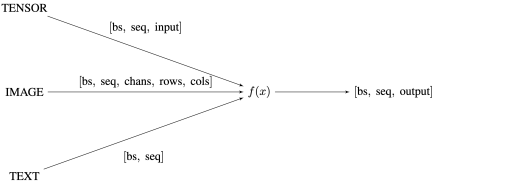Figure 6 / Standardization of input dimensions within the network

By working on abstract input classes we can ensure participants work towards a general multi-task understanding Kaiser et al. . Participants may use: (2) completely distinct computing substrates Nugent and Molter , (2) datasets Lample and Conneau , (3) models, and (4) strategies for maximizing their incentives in the market. It makes sense for peers to work on unsupervised datasets where data is cheap and privacy not required.

07 Conditional Computation

As the network grows, outward bandwidth is likely to become a major bottleneck. The need to reduce network transfer and a method of selecting peers is required. Conditional computation can be used where peers learn through gradient descent how to select and prune neighbors in the network. For example, a product key layer or a sparsely gated layer Shazeer et al. .

## (9)

$f_{i} = f_{i}(G(x))$

## (10)

$G(x) = \sum_{j} g_{j}(x) * f_{j}(x)$

The conditional layer determines a sparse combination of peers to query for each example and multiplicatively re-joins them, cutting outward bandwidth by querying only a small subset of peers for each example. The method can drastically increase outward bandwidth Shazeer et al.  Ryabinin and Gusev , allowing peers to communicate with many more neighbors in the graph. In essence, the layer acts as a trainable DNS lookup for peers based on inputs. Furthermore, being trainable with respect to the loss, it provides a useful proxy for the weights$\ w_{i,j} \in W \:$

08 Knowledge Extraction

Dependence between functions ensures that models must stay online and cannot be run in production. Breaking this dependence can be achieved using distillationHinton et al. : A compression and knowledge extraction technique in which a smaller model – the student - mimics the behaviour of the remaining network. The distillation layer is employed in conjunction with conditional computation (10) layer where the student model learns to mimic the network using the cross-entropy (shown below as KL) between the logits produced by the gating network and the student’s predicted distribution Sanh et al. .

## (11)

$\ distillation \ loss = KL_{D} (dist(x), G(x)$

Because the distilled model acts as a proxy for the network, models can be fully taken off-line and evaluated. Recursion through the network is also cut between components allowing for arbitrary network graphs. If models go offline, their peers can use the distilled versions in-place. Private data 6 can be validated over the distilled models instead of querying the network. Eventually, components can fully disconnect from the network using the distilled models to do validation and inference offline.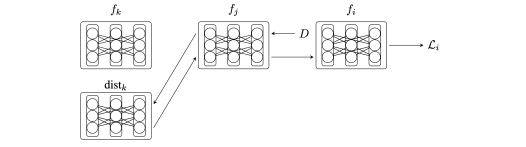Figure 7 / Queries propagate to depth=1 before the distilled model is used.

09 Learning Weights

Our goal in this work is the production of a ranking $\ r = [r_{i}] \:$over peers where score $\ r_{i} \in R$represents a participant’s information-theoretic significance to the benchmark. Following LeCun and others LeCun et al. ; Yu et al. , it is reasonable to define this significance by equating it with the cost of removing each peer from the network. We can derive this score analytically where$\ \Delta F(x)_{i} \:$is a perturbation of the$\ j^{th} \:$peers’s inputs when the,$\ i^{th} \:$peer is removed from the network (Appendix 12.2):

## (12)

$\ r_{i} \approx \frac{1}{n} \sum_{j}^{n} \sum_{x \in D_{j}} \Delta F^{T}(x)_{i} \cdot H(Q_{j}(x)) \cdot \Delta F(x)_{i} \\ \: \\ \Delta F(x)_{i} = [0, ...0,-f_{i}(x), 0, ...0]$

Note, when the error function$\ Q_{j} \:$is the twice-differentiable cross-entropy, then$\ H(Q_{j}) \:$is its Fisher- information matrix, and$\ r_{i} \in R \:$is suitably measured as each peer’s informational significance to the network as a whole. However, information theoretic weights require the full Hessian of the error. In practice it is more reasonable to use a heuristic to propagate a contribution score from the error function through to the inputs Yu et al. . For instance, weights from the gating layer (Section 6) provide a useful differentiable proxy.

10 Collusion

We consider the scenario where a subset of the peers in the network have formed a ’cabal’: A set of colluding peers attempting to maximize their inflation without accurately scoring their neighbors. The fight between the honest graph A with stake $\ S_{A}$ and the disjoint cabal B with stake $S_{B}$ can be determined by the proportion of network stake held by each. The honest graph must attain more inflation to maintain its dominance and protect the network$\ I_{A} \gg I_{B}$

We assume that the proportion of stake in the honest graph is more than that found in the dishonest graph$\ S_{A} \gg S_{B} \:$and that the chain has reached consensus$\ \mathcal{L} < 0 \:$Since all peers in B are disjoint from A, our loss term$\ -R_{B} \cdot (C_{B} - 0.5) > 0 \:$is positive. Because$\ \mathcal{L} < 0 \:$it must be the case that$\ R_{A} \cdot (C_{A} - 0.5) < 0 \:$is negative and there are peers in the honest sub-graph A who are connected to the majority.

As the chain progresses, newly minted stake is being emitted at our inflation rate τ in proportion to I = R · T. Importantly, the gradient of the incentive function with respect to the stake is positive and super-linear at our inflection point between the honest and dishonest graph. Notably,$\ \frac{\delta I}{\delta S} = \frac{5}{2}$, this ensures that the amount of stake held by each sub-graph reflect a non-linear change in their inflation at the next iteration.

Initially, since$\ S_{A} > 0.5 \: and \: S_{B} < 0.5$the proportion of stake emitted in sub-graph A exceeds that in sub-graph B, and sub-graph A’s incentive grows super-linearly compared to B. The result is that the ratio of stake$\ \frac{S_{B}}{S_{A} + S_{B}} \:$decreases – the cabal must continually add stake to its sub-graph to maintain itself through time.

We consider this proportion between the competing graphs under continuous inflation. Converting to python code ...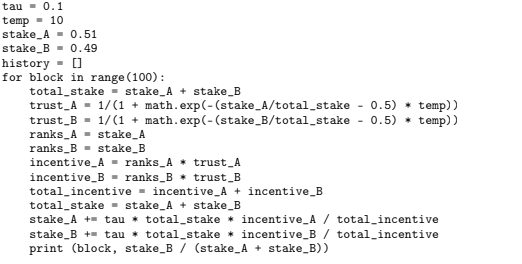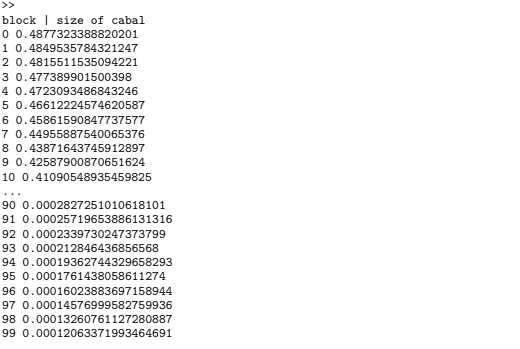11 Conclusion

We have proposed an intelligence market which runs on a P2P network outside of a trusted environ- ment. Crucially, the benchmark measures performance as representational-knowledge production using other intelligence systems to determine its value. The fact that this can be done in a collaborative and high-resolution manner suggests that the benchmark could provide a better reward mechanism for the field in general.

To achieve this aim, the paper began with the definition of a P2P network composed of abstractly defined intelligence models. We showed how this framework allowed us to produce a ranking for each peer based on the cost to prune it from the network. Peers negotiated this score using a set of weights on a digital ledger. However, the system was incomplete without mechanisms that prevented participants from forming dishonest sub-graphs.

To resolve this, we proposed an incentive scheme based on peer connectivity which exponentially rewarded peers for being trusted by a large portion of the network. This ensured that over time dishonest sub-graphs decay to irrelevance.

Following this, we showed (1) how peers reduced the network bandwidth by learning connectivity using a differential layer and (2) how they could extract fully network-disconnected machine learning models to run in production. The result is an intelligence market which rewards participants for producing knowledge and making it available to new learners in the system.